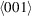International Tables for Crystallography (2006). Vol. A1, ch. 2.1, pp. 42-58   | 1 | 2 | https://doi.org/10.1107/97809553602060000542

## Contents

• 2.1. Guide to the subgroup tables and graphs  (pp. 42-58)
• 2.1.1. Contents and arrangement of the subgroup tables  (p. 42) | html | pdf |
• 2.1.2. Structure of the subgroup tables  (pp. 42-45) | html | pdf |
• 2.1.2.1. Headline  (p. 42) | html | pdf |
• 2.1.2.2. Data from IT A  (pp. 42-43) | html | pdf |
• 2.1.2.2.1. Generators selected  (p. 42) | html | pdf |
• 2.1.2.2.2. General position  (pp. 42-43) | html | pdf |
• 2.1.2.3. Specification of the setting  (p. 43) | html | pdf |
• 2.1.2.4. Sequence of the subgroup and supergroup data  (p. 43) | html | pdf |
• 2.1.2.5. Special rules for the setting of the subgroups  (pp. 43-45) | html | pdf |
• 2.1.2.5.1. Monoclinic subgroups  (p. 44) | html | pdf |
• 2.1.2.5.2. Subgroups with two origin choices  (pp. 44-45) | html | pdf |
• 2.1.2.5.3. Space groups with a rhombohedral lattice  (p. 45) | html | pdf |
• 2.1.3. I Maximal translationengleiche subgroups ( t -subgroups)  (pp. 45-48) | html | pdf |
• 2.1.3.1. Introduction  (p. 45) | html | pdf |
• 2.1.3.2. A description in close analogy with IT A  (pp. 45-46) | html | pdf |
• 2.1.3.3. Basis transformation and origin shift  (pp. 46-48) | html | pdf |
• 2.1.4. II Maximal klassengleiche subgroups ( k -subgroups)  (pp. 48-51) | html | pdf |
• 2.1.4.1. General description  (p. 48) | html | pdf |
• 2.1.4.2. Loss of centring translations  (pp. 48-49) | html | pdf |
• 2.1.4.3. Enlarged unit cell  (pp. 49-51) | html | pdf |
• 2.1.4.3.1. Enlarged unit cell, index 2  (pp. 49-50) | html | pdf |
• 2.1.4.3.2. Enlarged unit cell, index 3 or 4  (pp. 50-51) | html | pdf |
• 2.1.5. Series of maximal isomorphic subgroups  (pp. 51-53) | html | pdf |
• 2.1.5.1. General description  (p. 51) | html | pdf |
• 2.1.5.2. Basis transformation  (p. 51) | html | pdf |
• 2.1.5.3. Origin shift  (p. 51) | html | pdf |
• 2.1.5.4. Generators  (p. 52) | html | pdf |
• 2.1.5.5. Special series  (pp. 52-53) | html | pdf |
• 2.1.5.5.1. Monoclinic space groups  (p. 52) | html | pdf |
• 2.1.5.5.2. Trigonal space groups with rhombohedral lattice  (p. 52) | html | pdf |
• 2.1.5.5.3. Space groups with two origin choices  (pp. 52-53) | html | pdf |
• 2.1.6. Minimal supergroups  (pp. 53-54) | html | pdf |
• 2.1.6.1. General description  (p. 53) | html | pdf |
• 2.1.6.2. I Minimal translationengleiche supergroups  (p. 53) | html | pdf |
• 2.1.6.3. II Minimal non-isomorphic klassengleiche supergroups  (pp. 53-54) | html | pdf |
• 2.1.6.4. Isomorphic supergroups  (p. 54) | html | pdf |
• 2.1.7. The subgroup graphs  (pp. 54-58) | html | pdf |
• 2.1.7.1. General remarks  (p. 54) | html | pdf |
• 2.1.7.2. Graphs for translationengleiche subgroups  (pp. 54-55) | html | pdf |
• 2.1.7.3. Graphs for klassengleiche subgroups  (pp. 55-56) | html | pdf |
• 2.1.7.4. Graphs for plane groups  (pp. 56-57) | html | pdf |
• 2.1.7.5. Application of the graphs  (pp. 57-58) | html | pdf |
• References | html | pdf |
• Figures
• Fig. 2.1.7.1. Contracted graph of the group–subgroup chains from(225) to those subgroups with index 12 which belong to the space-group type(12)  (p. 57) | html | pdf |
• Fig. 2.1.7.2. Complete graph of the group–subgroup chains from(225) to one representative of those six(12) subgroups with index 12 whose monoclinic axes are along thedirections of(p. 57) | html | pdf |
• Fig. 2.1.7.3. Complete graph of the group–subgroup chains from(225) to one representative of those three(12) subgroups with index 12 whose monoclinic axes are along thedirections of(p. 57) | html | pdf |